Related Articles

# Compound Finite Automata (FA)

• Last Updated : 27 May, 2019

Prerequisite – Finite Automata (FA)
Compound FA is the resultant DFA formed after performing operation (∪, ∩, -) on given DFAs D1 and D2.

`D1 = (Q1, ∑, δ, q1, F1) and D2 = (Q2, ∑, δ, q2, F2) `

Where,
Q1 and Q2: Set of finite states of DFA D1 and D2 respectively.
∑: Input alphabet contain finite number of input symbols.
δ: Transition function (δ:Qx∑->Q)
q1 and q2: Initial state of D1 and D2 respectively.
F1 and F2: Set of final states of DFA D1 and D2 respectively.

Properties of Compound Finite Automata (FA):

1. Number of states in compound FA (D1XD2) is equal to m*n, where m is the number of states in D1 and n is the number of states D2.
2. Initial state of compound FA is combination of initial states of D1 and D2.
3. Final state of compound FA depends on the operation performed.

Example:

```D1 = no. of a's divisible by 2
D2 = no. of b's divisible by 3

D1 ({q1, B}, {a, b}, δ, q1, {q1}),
D1 ({q2, A, C}, {a, b}, δ, q2, {q2}) ```

Construct the minimal FA for:

```(D1∪D2),
(D1∩D2),
(D1-D2),
(D2-D1) ```

Explanation:

DFA D1: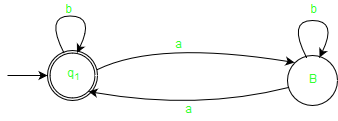DFA D2: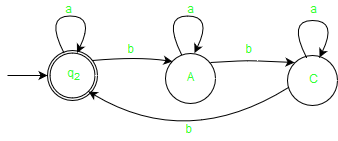1. Union (D1∪D2):
Any string w which is either belong’s to the language of D1 or the language of D2 is accepted by resultant compound automata.
Final state: If final state of D1 or final state of D2 contain in any of the states of compound FA.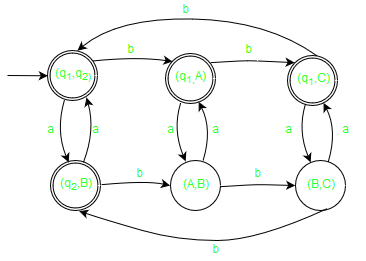2. Intersection (D1∩D2):
Any string w which belong’s to both the language of D1 and the language of D2 is accepted by resultant compound automata.
Final state: If both final state of D1 and final state of D2 contain in any of the states of compound FA.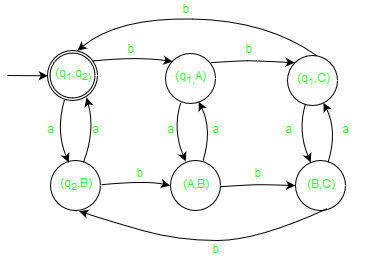3. Difference (D1 – D2):
Any string w which is accepted by D1, not by D2.
Final state: If final state of D1 and non-final state of D2 contain in any of the states of compound FA.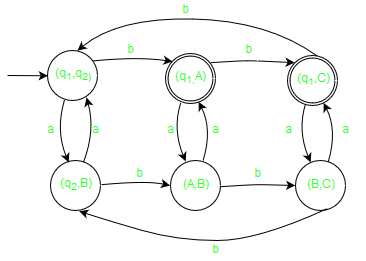4. Difference (D2 – D1):
Any string w which is accepted by D2, not by D1.
Final state: If final state of D2 and non-final state of D1 contain in any of the states of compound FA.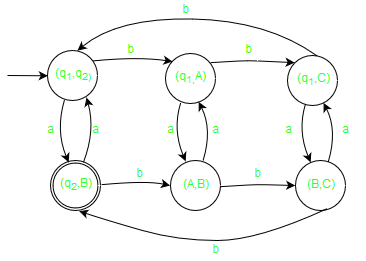Attention reader! Don’t stop learning now.  Practice GATE exam well before the actual exam with the subject-wise and overall quizzes available in GATE Test Series Course.

Learn all GATE CS concepts with Free Live Classes on our youtube channel.

My Personal Notes arrow_drop_up